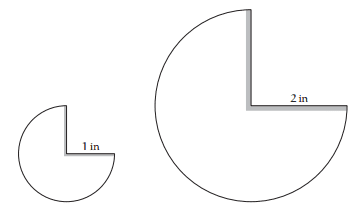# Drag Force on Paper Cones

Problem 2.14: Explaining the Simplification from Street-Fighting Mathematics by Mahajan
"Why is the drag force independent of the gravitational acceleration g and of the cone's mass m (yet the force depends on the cone's shape and size)?"

Context
Imagine two paper cones formed by taping together the edges of the cones shown in Figure 1 below. Both cones are similar in shape, but the larger cone has twice the height and width of the smaller cone. The cones are dropped point downward in the air. Mahajan proposes to "deduce the drag force as a function of fall speed and then to find the speed at which the drag balances the weight of the cones", thereby eliminating gravitational acceleration and cone mass.Figure 1. $\frac{3}{2}\pi$ sectors with radii 1" and 2", respectively

As an undergrad engineering student, I am familiar with seeing and applying the drag force equation
$F_d=\frac{1}{2}\rho u^2 C_D A$, but don't understand the physical basis for each of the terms present (and not present) in the formula. I would imagine that the drag force on a cone would be greater if a cone were dropped in an area of higher gravitational force (say, on Jupiter in a box filled with air similar in density to that on Earth). I could imagine that the velocity term is a function of something gravity-related, but don't fully understand why drag is independent of gravitational acceleration and mass. Please help me understand.

Attempt at a Solution 1
Revisiting some equations I remember deriving in general physics,
$a=const=g$
integrating with respect to time, $v=gt+v_0$
Those equations don't take friction into account, so I'm not sure how useful they are. At terminal velocity, velocity is constant and stops changing with respect to time. In that sense, i can see how g is no longer in the equation, but I would like to get a physical understanding of why this is true.

Attempt at a Solution 2
At terminal velocity, the net force on the falling object is zero. The only forces on the system are gravitational force ($F_g=mg$) and drag force. They must be equal and opposite in magnitude for the sum of net forces to be zero, but that would mean that drag is a function of both mass and gravitational acceleration. This is why I am confused.

*Note: this is "pleasure reading", not a school assignment. I've been stuck on this page for a week and would be grateful for help!

## Answers and Replies

Chestermiller
Mentor
Your drag equation determines the force that the air exerts on the body that is moving through it. So the focus is on the interaction between the air and the body. The density ρ is the density of the air and the velocity u is the velocity of the body relative to the air. The equation would apply if the body is falling through the air, but it would apply in exactly the same way if the body were stationary in a wind tunnel and the air were being blown past the body.

Let me guess: as an engineer, you haven't had a course in fluid mechanics yet, correct?

Chet

Your drag equation determines the force that the air exerts on the body that is moving through it. So the focus is on the interaction between the air and the body. The density ρ is the density of the air and the velocity u is the velocity of the body relative to the air. The equation would apply if the body is falling through the air, but it would apply in exactly the same way if the body were stationary in a wind tunnel and the air were being blown past the body.

Let me guess: as an engineer, you haven't had a course in fluid mechanics yet, correct?

Chet

I do understand that density and velocity are of the surrounding fluid (air) and not the object (the cone). I've actually taken transport phenomena as well as transport processes and a chemical engineering fundamentals laboratory course, so I am quite familiar with using and applying the equations. I have a poor understanding of the underlying physics, which bring me here. In other words, I know how and when to use the equation just fine. I don't understand why it is the way it is, which is something not often explored in my engineering classes.

Thanks for the wind tunnel example. I can see that gravitational acceleration and object mass don't show up in the equation. I'm still puzzled about why attempt 2 was wrong. Is it because drag in this case can be expressed in two different ways, one being mg and another being the drag equation we discussed?

Last edited:
Chestermiller
Mentor
The gravitational force and the drag force are two completely separate entities, resulting from two entirely different physical mechanisms. The gravitational force is caused by attraction of the earth on the cone, and would be present even if the drag force were zero (e.g., falling in a vacuum). The drag force is the result of viscous drag and air stagnation flow on the cone. This would be present even if there were no gravity (e.g., the wind tunnel example). The drag is expressed not "in two different" ways, but in only one way. In order for the drag force to match the force of gravity, the relative velocity of the cone with respect to the air has to attain a certain value to bring about this match. This velocity at this point is called the "terminal velocity."

Chet

A.T.
Science Advisor
I'm still puzzled about why attempt 2 was wrong.
The approach is fine to analyse the special case of falling at terminal velocity. But I have no idea why you think that the general drag formula should be based on this one special case.

Chet, that makes a lot of sense.

Thank you both for breaking it down for me.